安卓手机扫描二维码安装App

## 青羽讲数学史（四）

1.炎黄纪——鸿蒙太初诞生的灵智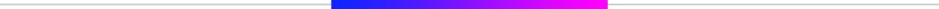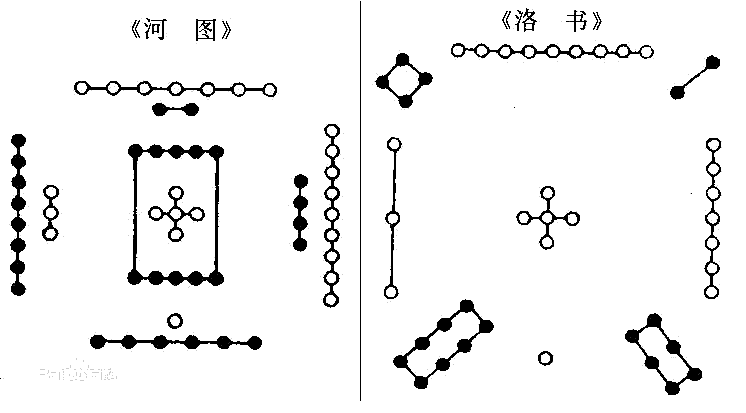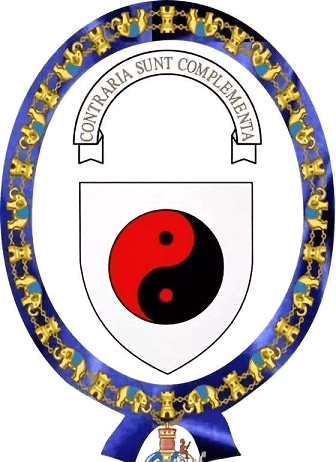2.春秋战国——百家争鸣中促成的数学体系《九章算术》不仅在后世近两千年的时间里作为中国历朝的贵族子弟的数学教材，还传至印度、阿拉伯、日本、朝鲜等国家，甚至通过这些地区远至欧洲，其影响力可见一般。有人说，《九章算术》之于东方，有如《几何原本》之于西方，这东西两颗明珠交相辉映，代表了两方数学体系的成熟。

3.魏晋朝——诘辩求胜中极速发展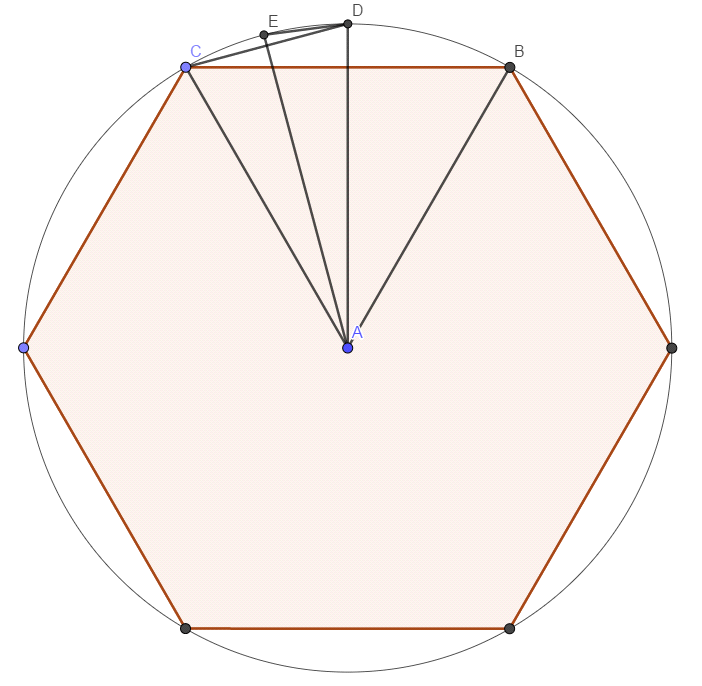﻿$r=1$﻿ ，则 S ﻿$= \pi r^2 = \pi$﻿

﻿$S_n$﻿ 表示正边形的面积，有 ﻿$\pi \approx S_n$﻿

﻿$S_6=6 S_{\triangle ABC}$﻿

﻿$=6 \times \dfrac{1}{2} \times 1 \times 1 \times sin 60 \degree$﻿

﻿$=3 sin 60 \degree$﻿

﻿$S_{12}=12S_{\triangle ACD}$﻿

﻿$=12 \times \dfrac{1}{2} \times 1 \times 1 \times sin 30 \degree$﻿

﻿$=6 sin 30 \degree$﻿

﻿$S_{24}=24S_{\triangle ADE }=12 sin 15 \degree$﻿

﻿$S_6 \approx 2.598$﻿﻿$S_{12} =3$﻿﻿$S_{24} \approx 3.106$﻿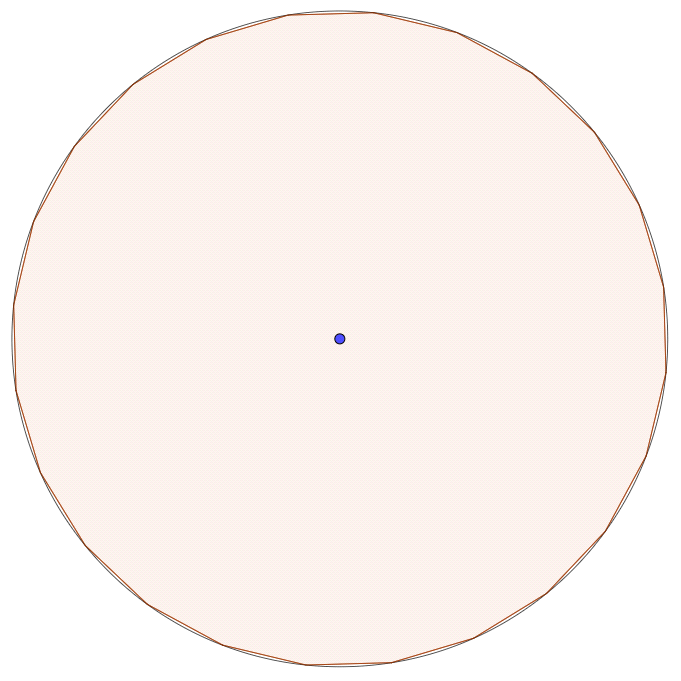﻿$S_{48}=24 sin 7.5 \degree$﻿ ，如何计算 ﻿$sin 7.5 \degree$﻿ 的值呢？

﻿$sin \dfrac{x}{2} = \sqrt{\dfrac{1-cosx}{2}}$﻿

﻿$=\sqrt{\dfrac{1-\sqrt{1-sin^2 x}}{2}}$﻿

﻿$\dfrac{360 \degree}{n} =x$﻿

﻿$\Rightarrow sin{\dfrac{x}{2}}= \sqrt{\dfrac{1-\sqrt{1-(\dfrac{2S_n}{n})^2}}{2}}$﻿

﻿$\therefore S_{2n}=nsin \dfrac{x}{2}$﻿

﻿$=\sqrt{\dfrac{n^2-n\sqrt{n^2 - 4 S_{n}^2}}{2}}$﻿

﻿$S_{5888} \approx 3.14159206$﻿

﻿$S_{11776} \approx 3.14159262$﻿

﻿$S_{94208} \approx 3.1415926513$﻿苹果手机扫描二维码安装App